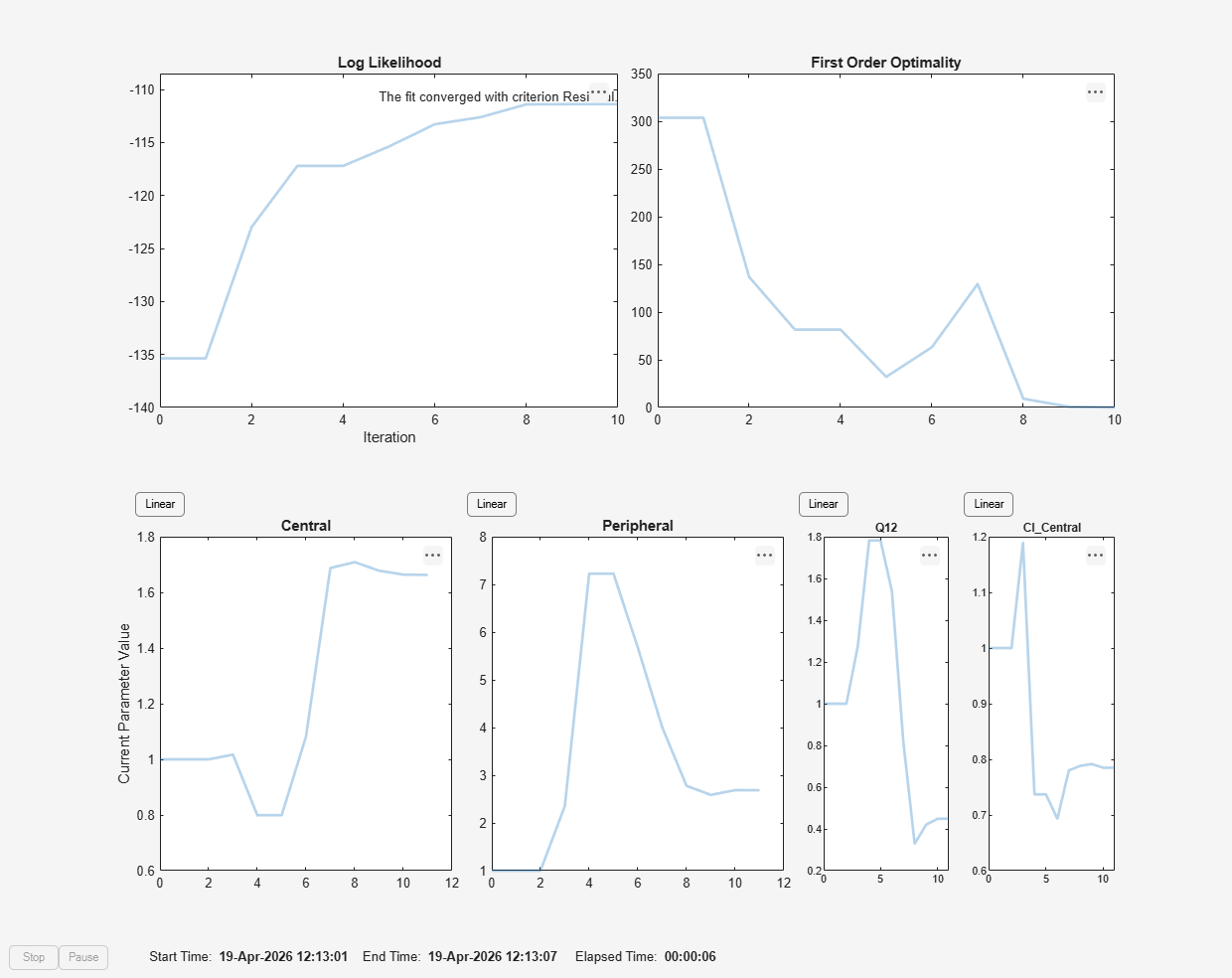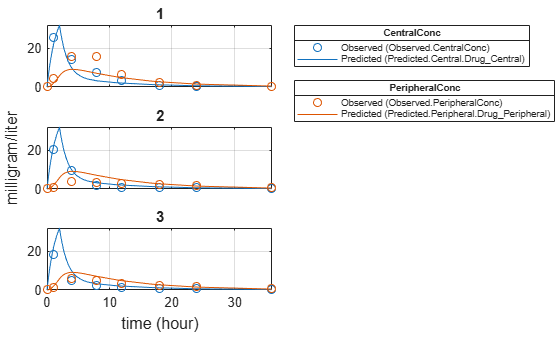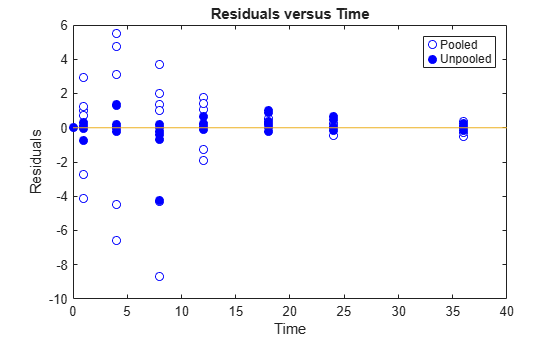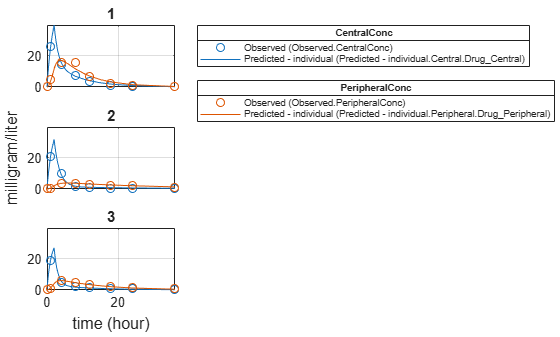# fitproblem

SimBiology problem object for parameter estimation

## Description

Create a `fitproblem` object to estimate model parameters using nonlinear regression or nonlinear mixed-effects modeling.

## Creation

Create the object using `fitproblem`.

expand all

## Required properties

Data to fit, specified as a `groupedData` object.

The name of the time variable must be defined in the `IndependentVariableName` property of `Data`. For instance, if the time variable name is `'TIME'`, then specify it as follows.

```prob = fitproblem; prob.Data = groupedData; prob.Data.TIME = [1:1:10]; prob.Data.Properties.IndependentVariableName = 'TIME';```

If the data contains more than one group of measurements, the grouping variable name must be defined in the `GroupVariableName` property of `Data`. For example, if the grouping variable name is `'GROUP'`, then specify it as follows.

`prob.Data.Properties.GroupVariableName = 'GROUP';`
A group usually refers to a set of measurements that represent a single time course, often corresponding to a particular individual or experimental condition.

Estimated parameters, specified as an `estimatedInfo` object, a vector of `estimatedInfo` objects, or a scalar `CovariateModel` object.

This property defines the estimated parameters in the model and other optional information such as their initial estimates, transformations, bound constraints, and categories. Supported transforms are `log`, `logit`, and `probit`. For details, see Parameter Transformations.

When you perform nonlinear regression by setting ```object.FitFunction = "sbiofit"```, then:

• Using `scattersearch` as an optimization function requires you to specify finite transformed bounds for each estimated parameter.

• If you do not specify the Pooled property, the software uses the `CategoryVariableName` property of the `estimatedInfo` object to decide if parameters must be estimated for each individual, group, category, or all individuals as a whole. Set the `Pooled` property to override any `CategoryVariableName` values. For details about the `CategoryVariableName` property, see `EstimatedInfo object`.

• The software uses the `categorical` function to identify groups. If any group values are converted to the same value by `categorical`, then those observations are treated as belonging to the same group. For instance, if some observations have no group information (that is, an empty character vector `''`), then `categorical` converts empty character vectors to `<undefined>`, and these observations are treated as one group.

For nonlinear mixed-effects modeling with `object.FitFunction="sbiofitmixed"`, the `CategoryVariablename` property of `estimatedInfo` object is ignored.

Name of a SimBiology estimation function to use, specified as `"sbiofit"` or `"sbiofitmixed"`. Use `sbiofit` for nonlinear regression problems and `sbiofitmixed` for nonlinear mixed-effects problems.

SimBiology model used to fit the data, specified as a `Model` object.

Mapping between model components and data variables, specified as a character vector, string, string vector, or cell array of character vectors.

Each character vector or string is an equation-like expression, similar to assignment rules. It contains the name (or qualified name) of a quantity (species, compartment, or parameter) or an `observable` object in the model, followed by the character `'='` and the name of a variable in `Data`. For clarity, white spaces are allowed between names and `'='`.

For example, if you have the concentration data `'CONC'` in `Data` for a species `'Drug_Central'`, you can specify it as follows.

`ResponseMap = 'Drug_Central = CONC';`

To name a species unambiguously, use the qualified name, which includes the name of the compartment. To name a reaction-scoped parameter, use the reaction name to qualify the parameter.

If the model component name or `grpData` variable name is not a valid MATLAB variable name, surround it by square brackets, such as:

`ResponseMap = '[Central 1].Drug = [Central 1 Conc]';`

If a variable name itself contains square brackets, you cannot use it in the expression to define the mapping information.

If any (qualified) name matches two components of the same type, an error is issued when you run the `fit` function of the object. To resolve the error, you can use a (qualified) name that matches two components of different types, and the function first finds the species with the given name, followed by compartments and then parameters.

Data Types: `char` | `string` | `cell`

## Optional properties

Doses applied during fitting, specified as an empty array or 2-D matrix of dose objects (`ScheduleDose` object or `RepeatDose` object). By default, the software applies no doses even if the model has active doses.

For a matrix of dose objects, it must have a single row or one row per group in the input data. If it has a single row, the same doses are applied to all groups during simulation. If it has multiple rows, each row is applied to a separate group, in the same order as the groups appear in the input data. Multiple columns are allowed so that you can apply multiple dose objects to each group.

For a cell vector of doses, it must have one element or one element per group in the input data. Each element must be `[]` or a vector of doses. Each element of the cell is applied to a separate group, in the same order as the groups appear in the input data.

In addition to manually constructing dose objects using `sbiodose`, if the input `groupedData` object has dosing information, you can use the `createDoses` method to construct doses.

Name of an optimization function that is called by `FitFunction` (`sbiofit` or `sbiofitmixed`), specified as a character vector or string.

If `FitFunction="sbiofit"`, valid choices are as follows.

• `"auto"`

• `"fminsearch"`

• `"nlinfit"` (Statistics and Machine Learning Toolbox™ is required.)

• `"fminunc"` (Optimization Toolbox™ is required.)

• `"fmincon"` (Optimization Toolbox is required.)

• `"lsqcurvefit"` (Optimization Toolbox is required.)

• `"lsqnonlin"` (Optimization Toolbox is required.)

• `"patternsearch"` (Global Optimization Toolbox is required.)

• `"ga"` (Global Optimization Toolbox is required.)

• `"particleswarm"` (Global Optimization Toolbox is required.)

• `"scattersearch"`

By default (that is, `FunctionName="auto"` and `FitFunction="sbiofit"`), the `fitproblem` object uses the first available estimation function among the following: `lsqnonlin` (Optimization Toolbox), `nlinfit` (Statistics and Machine Learning Toolbox), or `fminsearch`. The same priority applies to the default local solver choice for `scattersearch`.

If `FitFunction="sbiofitmixed"`, the valid choices are:

• `"auto"`

• `"nlmefit"`

• `"nlmefitsa"`

When `FunctionName="auto"` and `FitFunction="sbiofitmixed"`, the object uses `"nlmefit"` as the optimization function.

Data Types: `char` | `string`

Options for the optimization function, specified as a scalar struct, `optimoptions` object or empty array `[]`.

When `FitFunction="sbiofit"`, you can use the following options:

• `statset` struct for `nlinfit`

• `optimset` struct for `fminsearch`

• `optimoptions` object for `lsqcurvefit`, `lsqnonlin`, `fmincon`, `fminunc`, `particleswarm`, `ga`, and `patternsearch`

• `struct` for `scattersearch`

See Default Options for Optimization Functions Called by sbiofit for more details and default options associated with each estimation function.

When `FitFunction="sbiofitmixed"`, define a structure as follows:

• The structure can contain fields and default values that are the name-value arguments accepted by `nlmefit` (Statistics and Machine Learning Toolbox) and `nlmefitsa` (Statistics and Machine Learning Toolbox), except the following that are not supported.

• `'FEConstDesign'`

• `'FEGroupDesign`

• `'FEObsDesign'`

• `'FEParamsSelect'`

• `'ParamTransform'`

• `'REConstDesign'`

• `'REGroupDesign'`

• `'REObsDesign'`

• `'Vectorization'`

`'REParamsSelect'` is only supported when you provide a vector of `estimatedInfo` objects when specifying the estimated parameters.

• Use the `statset` (Statistics and Machine Learning Toolbox) function only to set the `'Options'` field of the structure (`opt`), as follows.

`opt.Options = statset('Display','iter','TolX',1e-3,'TolFun',1e-3);`

• For other supported name-value arguments (see `nlmefit` (Statistics and Machine Learning Toolbox) and `nlmefitsa` (Statistics and Machine Learning Toolbox)), set them as follows.

```opt.ErrorModel = 'proportional'; opt.ApproximationType = 'LME';```

Flag to show the progress of parameter estimation, specified as a numeric or logical `1` (`true`) or `0` (`false`). If the flag is `true`, a new figure opens containing plots during fitting.

When `FitFunction="sbiofit"`:

• Plots show the log-likelihood, termination criteria, and estimated parameters for each iteration. This option is not supported for `nlinfit`.

• If you are using the Optimization Toolbox functions (`fminunc`, `fmincon`, `lsqcurvefit`, `lsqnonlin`), the figure also shows the First Order Optimality (Optimization Toolbox) plot.

• For an unpooled fit, each line on the plots represents an individual. For a pooled fit, a single line represents all individuals. The line becomes faded when the fit is complete. The plots also keep track of the progress when you run `sbiofit` (for both pooled and unpooled fits) in parallel using remote clusters. For details, see Progress Plot.

When `FitFunction="sbiofitmixed"`, the plots show the values of fixed effects parameters (`theta`), the estimates of the variance parameters, that is, the diagonal elements of the covariance matrix of the random effects (Ψ), and the log-likelihood. For details, see Progress Plot.

Data Types: `double` | `logical`

Flag to enable parallelization, specified as a numeric or logical `1` (`true`) or `0` (`false`). If the flag is `true` and Parallel Computing Toolbox™ is available, the software enables the parallelization by doing the following:

1. Create `SimFunction` objects with `UseParallel=true`.

2. Pass the flag `UseParallel=true` to the optimization function, such as `lsqnonlin`. Note that some optimization functions do not support parallelization. See the reference page of the corresponding optimization function to find out about the parallelization support.

3. When `FitFunction="sbiofit"`, and you are performing an unpooled fit (`Pooled=false`) for multiple groups, each fit is run in parallel. For a pooled fit (`Pooled=true`), parallelization happens at the solver level. In other words, solver computations, such as objective function evaluations, are run in parallel.

Data Types: `double` | `logical`

Variants applied during fitting, specified as an empty array `[]` or a 2-D matrix or cell vector of variant objects. By default, the software applies no variants even if the model has active variants.

For a matrix of variant objects, the number of rows must be one or must match the number of groups in the input data. The ith row of variant objects is applied to the simulation of the ith group. The variants are applied in order from the first column to the last. If this matrix has only one row of variants, they are applied to all simulations.

For a cell vector of variant objects, the number of cells must be one or must match the number of groups in the input data. Each element must be `[]` or a vector of variants. If this cell vector has a single cell containing a vector of variants, they are applied to all simulations. If the cell vector has multiple cells, the variants in the ith cell are applied to the simulation of the ith group.

In addition to manually constructing variant objects using `sbiovariant`, if the input `groupedData` object has variant information, you can use `createVariants` to construct variants.

## Optional properties for `FitFunction="sbiofit"` only

Error models used for estimation, specified as a character vector, string, string vector, cell array of character vectors, categorical vector, or table.

If it is a table, it must contain a single variable that is a column vector of error model names. The names can be a cell array of character vectors, string vector, or a vector of categorical variables. If the table has more than one row, then the `RowNames` property must match the response variable names specified in the right hand side of `ResponseMap`. If the table does not use the `RowNames` property, the nth error is associated with the nth response.

If you specify only one error model, then `sbiofit` estimates one set of error parameters for all responses.

If you specify multiple error models using a categorical vector, string vector, or cell array of character vectors, the length of the vector or cell array must match the number of responses in `ResponseMap`.

You can specify multiple error models only if you are using these methods: `lsqnonlin`, `lsqcurvefit`, `fmincon`, `fminunc`, `fminsearch`, `patternsearch`, `ga`, and `particleswarm`.

Four built-in error models are available. Each model defines the error using a standard mean-zero and unit-variance (Gaussian) variable e, simulation results f, and one or two parameters a and b.

• `"constant"`: $y=f+ae$

• `"proportional"`: $y=f+b|f|e$

• `"combined"`: $y=f+\left(a+b|f|\right)e$

• `"exponential"`: $y=f\ast \mathrm{exp}\left(ae\right)$

Note

• If you specify an error model, you cannot specify weights except for the constant error model.

• If you use a proportional or combined error model during data fitting, avoid specifying data points at times where the solution (simulation result) is zero or the steady state is zero. Otherwise, you can run into division-by-zero problems. It is recommended that you remove those data points from the data set. For details on the error parameter estimation functions, see Maximum Likelihood Estimation.

Data Types: `double` | `char` | `string` | `table` | `cell`

Fit option flag to fit each individual or pool all individual data, specified as a numeric or logical `1` (`true`) or `0` (`false`), or `"auto"`.

If `Pooled` is set to `"auto"`, the software infers the value from the `Estimated` property as follows.

If `Estimated` is an `estimatedInfo` object with its `CategoryVariableName` property set to the default value `<GroupVariableName>`, then the `Pooled` property is set to `false`. Otherwise, the property is set to `true`.

• When the property is `false`, the `fit` function of the object estimates each group or individual separately using group- or individual-specific parameters, and the returned fit result is a vector of results objects with one result for each group.

• When the property is `true`, the `fit` function performs fitting for all individuals or groups simultaneously using the same parameter estimates, and the returned result is a scalar results object.

Note

Use this option to override the `CategoryVariableName` value of an `estimatedInfo` object.

Data Types: `char` | `string` | `double` | `logical`

Flag to use parameter sensitivities to determine gradients of the objective function, specified as a numeric or logical `1` (`true`) or `0` (`false`), or `"auto"`.

The default behavior (`"auto"`) is as follows.

• The property is `true` for `fmincon`, `fminunc`, `lsqnonlin`, `lsqcurvefit`, and `scattersearch` methods.

• Otherwise, the property is `false`.

If it is `true`, the software always uses the `sundials` solver, regardless of what you have selected as the `SolverType` property in the `Configset` object.

The software uses the complex-step approximation method to calculate parameter sensitivities. Such calculated sensitivities can be used to determine gradients of the objective function during parameter estimation to improve fitting. The default behavior of `sbiofit` is to use such sensitivities to determine gradients whenever the algorithm is gradient-based and if the SimBiology model supports sensitivity analysis. For details about the model requirements and sensitivity analysis, see Sensitivity Analysis in SimBiology.

Data Types: `double` | `logical` | `char` | `string`

Weights used for fitting, specified as an empty array `[]`, matrix of real positive weights where the number of columns corresponds to the number of responses, or a function handle that accepts a vector of predicted response values and returns a vector of real positive weights.

If you specify an error model, you cannot use weights except for the constant error model. If neither the `ErrorModel` or `Weights` is specified, by default, the software uses the constant error model with equal weights.

Data Types: `double` | `function_handle`

## Object Functions

 `fit` Perform parameter estimation using SimBiology problem object `resetoptions` Reset optional SimBiology fit problem properties

## Examples

collapse all

This example shows how to estimate PK parameters of a SimBiology model using a problem-based approach.

Load a synthetic data set. It contains drug plasma concentration data measured in both central and peripheral compartments.

`load('data10_32R.mat')`

Convert the data set to a `groupedData` object.

```gData = groupedData(data); gData.Properties.VariableUnits = ["","hour","milligram/liter","milligram/liter"];```

Display the data.

```sbiotrellis(gData,"ID","Time",["CentralConc","PeripheralConc"],... Marker="+",LineStyle="none");```Use the built-in PK library to construct a two-compartment model with infusion dosing and first-order elimination. Use the configset object to turn on unit conversion.

```pkmd = PKModelDesign; pkc1 = addCompartment(pkmd,"Central"); pkc1.DosingType = "Infusion"; pkc1.EliminationType = "linear-clearance"; pkc1.HasResponseVariable = true; pkc2 = addCompartment(pkmd,"Peripheral"); model2cpt = construct(pkmd); configset = getconfigset(model2cpt); configset.CompileOptions.UnitConversion = true;```

Assume every individual receives an infusion dose at time = 0, with a total infusion amount of 100 mg at a rate of 50 mg/hour. For details on setting up different dosing strategies, see Doses in SimBiology Models.

```dose = sbiodose("dose","TargetName","Drug_Central"); dose.StartTime = 0; dose.Amount = 100; dose.Rate = 50; dose.AmountUnits = "milligram"; dose.TimeUnits = "hour"; dose.RateUnits = "milligram/hour";```

Create a problem object.

`problem = fitproblem`
```problem = fitproblem with properties: Required: Data: [0x0 groupedData] Estimated: [1x0 estimatedInfo] FitFunction: "sbiofit" Model: [0x0 SimBiology.Model] ResponseMap: [1x0 string] Optional: Doses: [0x0 SimBiology.Dose] FunctionName: "auto" Options: [] ProgressPlot: 0 UseParallel: 0 Variants: [0x0 SimBiology.Variant] sbiofit options: ErrorModel: "constant" Pooled: "auto" SensitivityAnalysis: "auto" Weights: [] ```

Define the required properties of the object.

```problem.Data = gData; problem.Estimated = estimatedInfo(["log(Central)","log(Peripheral)","Q12","Cl_Central"],InitialValue=[1 1 1 1]); problem.Model = model2cpt; problem.ResponseMap = ["Drug_Central = CentralConc","Drug_Peripheral = PeripheralConc"];```

Define the dose to be applied during fitting.

`problem.Doses = dose;`

Show the progress of the estimation.

`problem.ProgressPlot = true;`

Fit the model to all of the data pooled together: that is, estimate one set of parameters for all individuals by setting the `Pooled` property to `true`.

`problem.Pooled = true;`

Perform the estimation using the `fit` function of the object.

`pooledFit = fit(problem);`Display the estimated parameter values.

`pooledFit.ParameterEstimates`
```ans=4×3 table Name Estimate StandardError ______________ ________ _____________ {'Central' } 1.6627 0.16569 {'Peripheral'} 2.6864 1.0644 {'Q12' } 0.44945 0.19943 {'Cl_Central'} 0.78497 0.095621 ```

Plot the fitted results.

`plot(pooledFit);`Estimate one set of parameters for each individual and see if the parameter estimates improve.

```problem.Pooled = false; unpooledFit = fit(problem);```Display the estimated parameter values.

`unpooledFit.ParameterEstimates`
```ans=4×3 table Name Estimate StandardError ______________ ________ _____________ {'Central' } 1.422 0.12334 {'Peripheral'} 1.5619 0.36355 {'Q12' } 0.47163 0.15196 {'Cl_Central'} 0.5291 0.036978 ```
```ans=4×3 table Name Estimate StandardError ______________ ________ _____________ {'Central' } 1.8322 0.019672 {'Peripheral'} 5.3364 0.65327 {'Q12' } 0.2764 0.030799 {'Cl_Central'} 0.86035 0.026257 ```
```ans=4×3 table Name Estimate StandardError ______________ ________ _____________ {'Central' } 1.6657 0.038529 {'Peripheral'} 5.5632 0.37063 {'Q12' } 0.78361 0.058657 {'Cl_Central'} 1.0233 0.027311 ```
`plot(unpooledFit);`Generate a plot of the residuals over time to compare the pooled and unpooled fit results. The figure indicates unpooled fit residuals are smaller than those of the pooled fit, as expected. In addition to comparing residuals, other rigorous criteria can be used to compare the fitted results.

```t = [gData.Time;gData.Time]; res_pooled = vertcat(pooledFit.R); res_pooled = res_pooled(:); res_unpooled = vertcat(unpooledFit.R); res_unpooled = res_unpooled(:); figure; plot(t,res_pooled,"o",MarkerFaceColor="w",markerEdgeColor="b") hold on plot(t,res_unpooled,"o",MarkerFaceColor="b",markerEdgeColor="b") refl = refline(0,0); % A reference line representing a zero residual title("Residuals versus Time"); xlabel("Time"); ylabel("Residuals"); legend(["Pooled","Unpooled"]);```As illustrated, the unpooled fit accounts for variations due to the specific subjects in the study, and, in this case, the model fits better to the data. However, the pooled fit returns population-wide parameters. As an alternative, if you want to estimate population-wide parameters while considering individual variations, you can perform nonlinear mixed-effects (NLME) estimation by setting `problem.FitFunction` to `sbiofitmixed`.

`problem.FitFunction = "sbiofitmixed";`
`NLMEResults = fit(problem);`Display the estimated parameter values.

`NLMEResults.IndividualParameterEstimates`
```ans=12×3 table Group Name Estimate _____ ______________ ________ 1 {'Central' } 1.4623 1 {'Peripheral'} 1.5306 1 {'Q12' } 0.4587 1 {'Cl_Central'} 0.53208 2 {'Central' } 1.783 2 {'Peripheral'} 6.6623 2 {'Q12' } 0.3589 2 {'Cl_Central'} 0.8039 3 {'Central' } 1.7135 3 {'Peripheral'} 4.2844 3 {'Q12' } 0.54895 3 {'Cl_Central'} 1.0708 ```

Plot the fitted results.

`plot(NLMEResults);`Plot the conditional weighted residuals (CWRES) and individual weighted residuals (IWRES) of model predicted values.

`plotResiduals(NLMEResults,'predictions')`